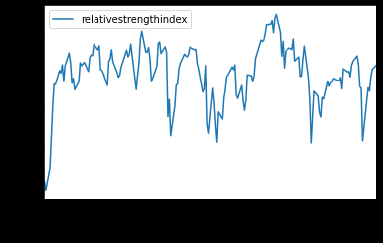# QuantBook Analysis Tool
qb = QuantBook()
#history = qb.History(qb.Securities.Keys, 360, Resolution.Daily)

# Create a 14 period RSI indicator
rsi = RelativeStrengthIndex(14)

df_rsi = qb.Indicator(rsi, spy.Symbol, 180, Resolution.Daily)
df_rsi.drop(['averageloss', 'averagegain'], axis=1, inplace=True).plot()
---------------------------------------------------------------------------
AttributeError                            Traceback (most recent call last)
<ipython-input-48-07024c2ec349> in <module>
10
11 df_rsi = qb.Indicator(rsi, spy.Symbol, 180, Resolution.Daily)
---> 12 df_rsi.drop(['averageloss', 'averagegain'], axis=1, inplace=True).plot()
13
14

AttributeError: 'NoneType' object has no attribute 'plot'

It works if I change inplace=False.Why?

Where to find reference on setting inplace value in drop method?

Thanks